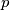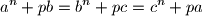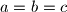### IMO Shortlist 2008 problem N1

Kvaliteta:
Avg: 0,0
Težina:
Avg: 6,5
Let$n$ be a positive integer and let$p$ be a prime number. Prove that if$a$,$b$,$c$ are integers (not necessarily positive) satisfying the equationsthen$a = b = c$.

Proposed by Angelo Di Pasquale, Australia
Izvor: Međunarodna matematička olimpijada, shortlist 2008